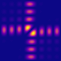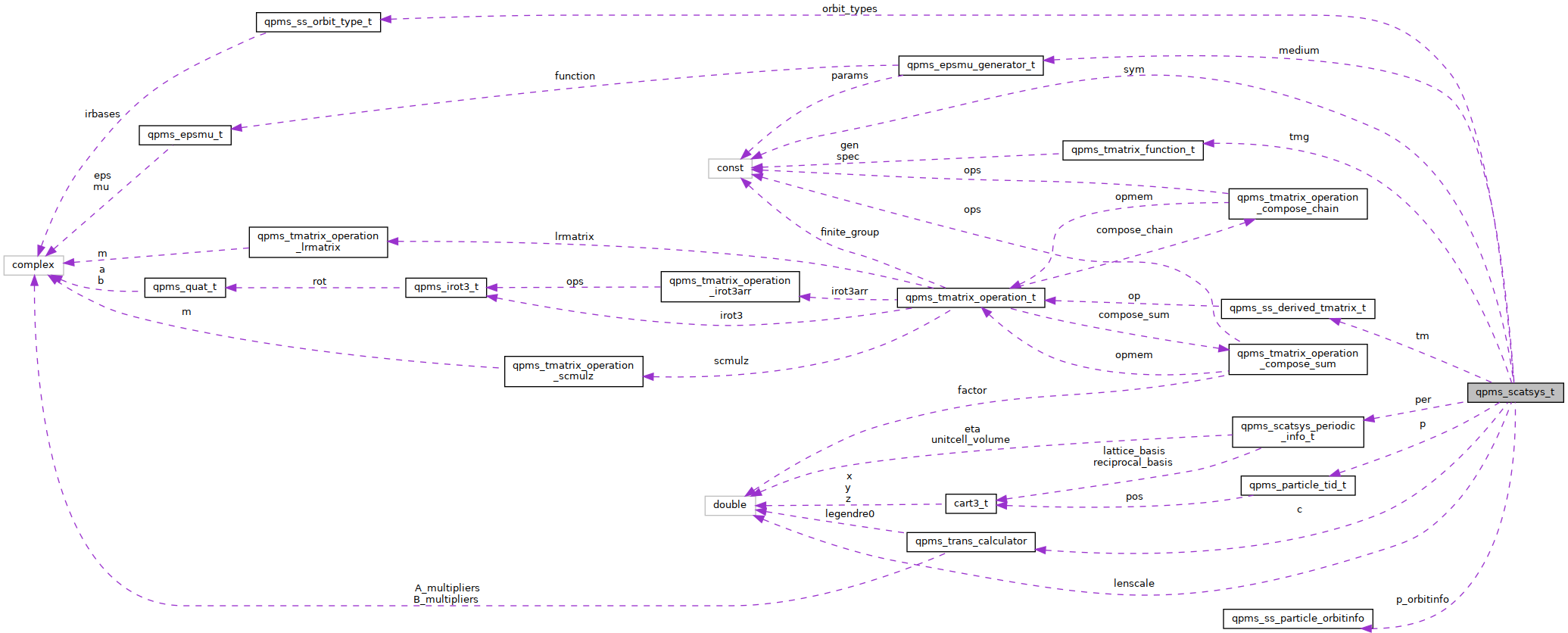QPMS Electromagnetic multiple scattering library and toolkit.
qpms_scatsys_t Struct Reference

Common "class" for system of scatterers, both periodic and non-periodic. More...

#include <scatsystem.h>

Collaboration diagram for qpms_scatsys_t:[legend]

## Data Fields

int lattice_dimension
Number of dimensions in which the system is periodic from the range 0–3.

struct qpms_epsmu_generator_t medium
Optical properties of the background medium.

qpms_tmatrix_function_ttmg
(Template) T-matrix functions in the system. More...

qpms_ss_tmgi_t tmg_count
Number of all different original T-matrix generators in the system.

qpms_ss_derived_tmatrix_ttm
All the different T-matrix functions in the system, including those derived from tmg elements by symmetries.

qpms_ss_tmi_t tm_count
Number of all different T-matrices in the system (length of tm[]).

qpms_ss_tmi_t tm_capacity
Capacity of tm[].

qpms_particle_tid_tp
Particles.

qpms_ss_pi_t p_count
Size of particles array.

qpms_ss_pi_t p_capacity
Capacity of p[].

const struct qpms_finite_group_tsym
Symmetry group of the array.

qpms_ss_pi_tp_sym_map
p_sym_map[idi + pi * sym->order] gives the index of pi-th particle under the idi'th sym op. More...

qpms_ss_tmi_ttm_sym_map
Which t-matrices map onto which by the symmetry ops. Lookup by tm_sum_map[idi + tmi * sym->order].

qpms_ss_oti_t orbit_type_count

qpms_ss_orbit_type_torbit_types
(Array length is orbit_type_count.)

qpms_ss_particle_orbitinfo_tp_orbitinfo
Orbit type identification of each particle. (Array length is p_count.)

size_t fecv_size
Number of elements of a full excitation coefficient vector size.

size_t * saecv_sizes
Number of elements of symmetry-adjusted coefficient vector sizes (order as in sym->irreps).

size_t * fecv_pstarts
Indices of where pi'th particle's excitation coeffs start in a full excitation coefficient vector.

size_t * saecv_ot_offsets
TODO DOC. In the packed vector, saecv_ot_offsets[iri * orbit_type_count + oti] indicates start of ot. More...

size_t max_bspecn
‍**< First index is irrep index as in sym->irreps, second index is particle index. *‍/ More...

qpms_ss_pi_tp_by_orbit
Particles grouped by orbit (in the order corresponding to the packed memory layout).

char * otspace

char * otspace_end

double lenscale

struct qpms_trans_calculatorc

qpms_scatsys_periodic_info_t per

## Detailed Description

Common "class" for system of scatterers, both periodic and non-periodic.

Infinite periodic structures (those with lattice_dimension > 0) have the per filled. These are ignored for finite systems (lattice_dimension == 0).

## ◆ max_bspecn

 size_t qpms_scatsys_t::max_bspecn

‍**< First index is irrep index as in sym->irreps, second index is particle index. *‍/

Maximum tm[...]->spec->n. Mainly for workspace allocation.

## ◆ p_sym_map

 qpms_ss_pi_t* qpms_scatsys_t::p_sym_map

p_sym_map[idi + pi * sym->order] gives the index of pi-th particle under the idi'th sym op.

Which particles map onto which by the symmetry ops.

## ◆ saecv_ot_offsets

 size_t* qpms_scatsys_t::saecv_ot_offsets

TODO DOC. In the packed vector, saecv_ot_offsets[iri * orbit_type_count + oti] indicates start of ot.

TODO maybe move it to qpms_ss_orbit_type_t, ffs.

## ◆ tmg

 qpms_tmatrix_function_t* qpms_scatsys_t::tmg

(Template) T-matrix functions in the system.

The qpms_abstract_tmatrix_t objects (onto which this array member point) are NOT owned by this and must be kept alive for the whole lifetime of all qpms_scatsys_t objects that are built upon them.

The documentation for this struct was generated from the following file: